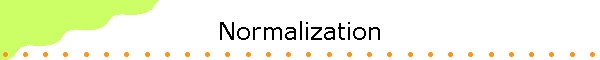In-class group activity 7:  Normalizing a wave function

Open a Microsoft Word document to keep a log of your results and conclusions.

The wave function ψ(x,0) = cos(x) for x between -π/2 and +π/2 and ψ(x) = 0 for all other x can be normalized.
You can show this by opening the linked spreadsheet
It has a column for x an a column for ψ(x,0) = N*cos(x) for x between -π and +π with N = 1 initially.  The maximum value of ψ(x,0) is 1.

• Add a column (column C) which contains |ψ(x,0)|2.  Since ψ(x,0) is a real function at t = 0, |ψ(x,0)|2 is just the square of the function.
• Now find ∑|ψ(xi,t)|2Δxi, i.e. find the area under the curve.
Into cell D2 type =C2*(A3-A2).  Into cell D3 type =D2+C3*(A4-A3).  Copy this formula down to row 81.
• You have just numerically integrated the function |ψ(x,0)|2.  The numerical value in row 81 is the integral.  It is not equal to 1.
To normalize ψ(x,0) we divide |ψ(x)|2 by the number stored in cell D81, or we divide ψ(x,0) by the square root of this number.
This can easily be accomplished by making N equal to 1 divided by the square root of the number stored in cell D81.
Highlight cell D81 and click edit, copy on Excel's menu bar.
Highlight  cell L19 and click edit, paste special, values.
This copies the value of cell D81 into cell L19.  (This step avoids a circular reference error in Excel.)
Into cell L20 type =1/SQRT(L19).
Excel will now normalize the wave function by multiplying it by the number stored in cell L20.
• Inspect the result and discuss it with your neighbors.
What is the new maximum value of ψ(x,0)?
What happened to the integral of |ψ(x,0)|2, the value stored in cell D81?
Does it confirm that the function is normalized?
Assume ψ(x,0) is the wave function of an electron at t = 0.  What is the probability of finding the electron in the small interval Δx between x = - 1 and x = -0.95?  What is the probability of finding it in the small interval Δx between x = 0 and x = 0.05?